Next: Selecting a Target Histogram Up: Shading and Lighting Normalization Previous: Shading and Lighting Normalization

## Histogram Fitting

Let H(i) be the histogram function of an image, and let G(i) be the desired histogram we wish to map to via a transfer function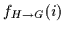. First, we compute a transfer function for both H(i) and G(i)that will map the histogram to a uniform distribution histogram, U(i). The functions are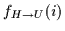and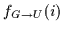respectively. Equationand Equationdepict the mapping to a uniform distribution which is also known as histogram equalization :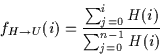(4.20)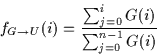(4.21)

where n is the number of discrete intensity levels, for 8-bit images, n=256.

To find the mapping function,, we invert the functionto obtain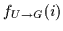. Since the domain and the range of the functions of this form are identical, the inverse mapping is trivial and is found by cycling through all values of the function. However, due to the discrete nature of these functions, inverting can yield a function which is undefined for certain values. Thus, we use linear interpolation and assume smoothness to fill undefined points of the inverse function according to the values of well-defined points in the function. Thus, we generate a fully defined mappingwhich transforms a uniform histogram distribution to the distribution found in histogram G(i). The mappingcan then be defined as in Equation: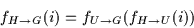(4.22)Next: Selecting a Target Histogram Up: Shading and Lighting Normalization Previous: Shading and Lighting Normalization
Tony Jebara
2000-06-23